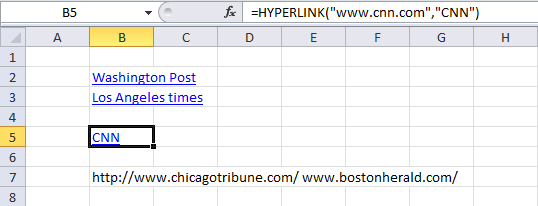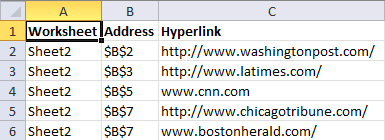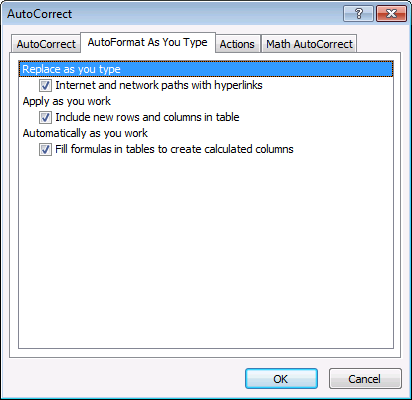Author: Oscar Cronquist Article last updated on April 21, 2021The macro in this blog post lists all hyperlinks in a worksheet. It also lists all words beginning with "http" or "www". It grabs the hyperlink even if you have a hyperlink function in a cell.

Example, this sheet has two regular hyperlinks (B2:B3) and one hyperlink function (B5) and two web addresses (B7).

This is what the macro returns, the worksheet name in column A. Cell address in column B and the hyperlink in column C.## 1. VBA Macro- list all hyperlinks in the worksheet

```'Name macro

'The SET statement allows you to save an object reference to a variable
'Save activesheet to object Asheet
Set Asheet = ActiveSheet

'Insert a new worksheet and save to object Nsheet

'Change to bold font
Nsheet.Range("A1:C1").Font.Bold = True

'Save 0 (zero) to variable i
i = 0

'Iterate through each cell in UsedRange
'UsedRange returns a Range object that represents the used range on the specified worksheet.
For Each cell In Asheet.UsedRange

'Enable error handling
On Error Resume Next

'Check if cell has a hyperlink and save the hyperlink to variable lnk

'Check if no error has occurred
If Err = 0 Then

'Save worksheet name to column A based on variable i
Nsheet.Range("A2").Offset(i, 0) = Asheet.Name

'Save cell address to column B based on variable i

'Add 1 to variable i and save result to variable i
i = i + 1

'Go here if an error has occurred
Else

'Check if formula begins with "=HYPERLINK("
If Left(cell.Formula, 11) = "=HYPERLINK(" Then

'Split formula and save to variable strArray
strArray = Split(cell.Formula, Chr(34))

'Save worksheet name to column A based on variable i
Nsheet.Range("A2").Offset(i, 0) = Asheet.Name

'Save cell address to column B based on variable i

Nsheet.Range("C2").Offset(i, 0) = strArray(1)

'Add 1 to variable i and save result to variable i
i = i + 1

'Go here if formulas does not begin with "=HYPERLINK("
Else

'Split cell into an array based on default delimiting value (space character) and save to array variable strArray
strArray = Split(cell)

'Iterate through each value in array strArray
For Each vl In strArray

'Check if array value is equal to http or www
If Left(vl, 4) = "http" Or Left(vl, 3) = "www" Then

'Save worksheet name to column A based on variable i
Nsheet.Range("A2").Offset(i, 0) = Asheet.Name

'Save cell address to column B based on variable i

Nsheet.Range("C2").Offset(i, 0) = vl

'Add 1 to variable i and save result to variable i
i = i + 1
End If
Next vl
End If
End If

'Disable error handling
On Error GoTo 0
Next cell

'Change column widths for A:C
Nsheet.Columns("A:C").AutoFit

End Sub
```

## 2. Where to put the code?

1. Press Alt +F11 to open the Visual Basic Editor (VBE).
2. Press with left mouse button on "Insert" on the top menu.
3. Press with mouse on "Module" to insert a module.
4. Paste VBA code to code window.
5. Exit VB Editor Alt + Q.

Save workbook with file extension *.xlsm (macro-enabled workbook).

## 3. How to start macro?

1. Press Alt+F8, a dialog box appears.
2. Press with left mouse button on "ListHyperlinks" to select the macro name.
3. Press with left mouse button on "Run" button to run the selected macro.

## 4. What happens when I run the macro?

1. A new worksheet is inserted.
2. The worksheet is populated with hyperlink data.
3. Macro ends.

## 5. How do I find all hyperlinks in a workbook?

This macro allows you to find links in all worksheets in the active workbook.

```Sub ListHyperlinksInWB()

Nsheet.Range("A1:C1").Font.Bold = True

i = 0
For Each sh In ActiveWorkbook.Worksheets
If sh.Name <> Nsheet.Name Then

For Each cell In sh.UsedRange
On Error Resume Next
If Err = 0 Then
Nsheet.Range("A2").Offset(i, 0) = sh.Name
i = i + 1
Else
If Left(cell.Formula, 11) = "=HYPERLINK(" Then
strArray = Split(cell.Formula, Chr(34))
Nsheet.Range("A2").Offset(i, 0) = sh.Name
Nsheet.Range("C2").Offset(i, 0) = strArray(1)
i = i + 1
Else
strArray = Split(cell)
For Each vl In strArray
If Left(vl, 4) = "http" Or Left(vl, 3) = "www" Then
Nsheet.Range("A2").Offset(i, 0) = sh.Name
Nsheet.Range("C2").Offset(i, 0) = vl
i = i + 1
End If
Next vl
End If
End If
On Error GoTo 0
Next cell
End If
Next sh

Nsheet.Columns("A:C").AutoFit

End Sub

```

## 6. How to disable automatic hyperlinking in Excel?

Tip! If you want to stop automatic hyperlinking (Excel 2010) follow these steps:

1. Press with left mouse button on "File" on the ribbon
2. Press with left mouse button on "Options"
3. Press with left mouse button on "Proofing"
4. Press with left mouse button on "AutoCorrect Options..."
5. Go to "Autoformat as you type" on the menu
6. Disable "Internet and network paths with hyperlinks"